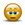# Scatter Pot with Linear Regression Trend

## Excel Help for Scatter Pot With Linear Regression Trend in Analyzing Corporate Financial DataIn this case, you can start by creating a simple XY scatter plot chart from the source data. By selecting the series in the chart you can right click and select Add Trend line > Linear.  Right click the Trend Line and choose Form Trend line > Options and choose to add the equation and R-squared on the chart.  This will give you the regression equation and the predictive quality (R-squared) for your analysis.To transform or analyze the data before charting, you can use the LOG function and/or other statistical functions such as SLOPE (regression coefficient) under the Statistical functions in Excel. Excel Business Forums Administrator Posted by Excel Helper on 25 Sep 2010